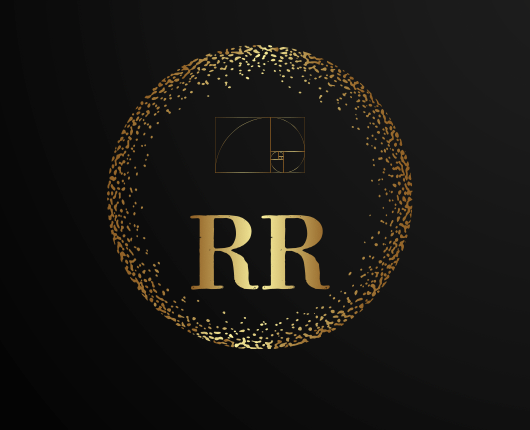# Logo RR & new Digital Catalog availableNew Logo is inspired by the Fibonacci sequence.

In mathematics, the Fibonacci numbers, commonly denoted Fn, form a sequence, called the Fibonacci sequence, such that each number is the sum of the two preceding ones, starting from 0 and 1. That is,

F0=0,F1=1,and

Fn=Fn−1+Fn−2,for n > 1.

The beginning of the sequence is thus:

0,1,1,2,3,5,8,13,21,34,55,89,144,…In some older books, the value F0=0is omitted, so that the sequence starts with F1=F2=1,and the recurrence Fn=Fn−1+Fn−2is valid for n > 2.The Fibonacci spiral: an approximation of the golden spiral created by drawing circular arcs connecting the opposite corners of squares in the Fibonacci tiling; (see preceding image)

Fibonacci numbers are strongly related to the golden ratio: Binet’s formula expresses the nth Fibonacci number in terms of n and the golden ratio, and implies that the ratio of two consecutive Fibonacci numbers tends to the golden ratio as n increases.

Fibonacci numbers are named after Italian mathematician Leonardo of Pisa, later known as Fibonacci. In his 1202 book Liber Abaci, Fibonacci introduced the sequence to Western European mathematics, although the sequence had been described earlier in Indian mathematics, as early as 200 BC in work by Pingala on enumerating possible patterns of Sanskrit poetry formed from syllables of two lengths.

Fibonacci numbers appear unexpectedly often in mathematics, so much so that there is an entire journal dedicated to their study, the Fibonacci Quarterly. Applications of Fibonacci numbers include computer algorithms such as the Fibonacci search technique and the Fibonacci heap data structure, and graphs called Fibonacci cubes used for interconnecting parallel and distributed systems.

They also appear in biological settings, such as branching in trees, the arrangement of leaves on a stem, the fruit sprouts of a pineapple, the flowering of an artichoke, an uncurling fern, and the arrangement of a pine cone’s bracts.New digital catalog available, please click on the logo above or here.

Enjoy the new online presentation,
Ramona Romanu

1 Comments for : Logo RR & new Digital Catalog available
•Ramona Romanu
• 3. April 2022